Normal view

# A SAS/IML companion for linear models / Jamis J. Perrett.

Material type:TextLanguage: English Series: Statistics and computingPublication details: c2010. Description: xiv, 228 p. : ill. ; 24 cmISBN: 9781441955562 (pbk. : acidfree paper); 1441955569 (pbk. : acidfree paper); 1441955577 (ebk.); 9781441955579 (ebk.)DDC classification: 519.535 LOC classification: QA276.4 | .P468 2010Online resources: WorldCat details | E-book Fulltext
Contents:
TOC SAS/IML: A Brief Introduction.- IML Language Structure.- IML Programming Features.- Matrix Manipulations in SAS/IML.- Mathematical and Statistical Basics.- Linear Algebra.- The Multivariate Normal Distribution.- The General Linear Model.- Linear Mixed Models.- Statistical Computation Methods.
Summary: Linear Models Courses are often presented as either theoretical or applied. This book bridges the gap between the derivation of formulas and analyses that hide these formulas. It includes complete examples of SAS code as well as code for high-level procedures.
Tags from this library: No tags from this library for this title.
Star ratingsAverage rating: 0.0 (0 votes)
Holdings
Item type Current library Collection Call number Copy number Status Date due Barcode Item holdsE-Book
E-book
Non-fiction 519.535 PES 2010 (Browse shelf(Opens below)) Not for loanText
Reserve Section
Non-fiction 519.535 PES 2010 (Browse shelf(Opens below)) C-1 Not For Loan 26642
Total holds: 0
##### Browsing EWU Library shelves, Shelving location: E-book Close shelf browser (Hides shelf browser)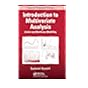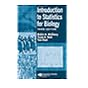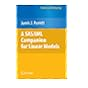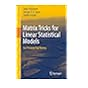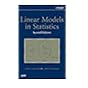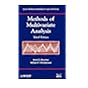519.535 KOI 2014 Introduction to multivariate analysis : 519.535 MCI 2007 Introduction to statistics for biology / 519.535 NEI 2006 An introduction to copulas / 519.535 PES 2010 A SAS/IML companion for linear models / 519.535 PUM 2011 Matrix tricks for linear statistical models : 519.535 REL 2008 Linear models in statistics / 519.535 REM 2012 Methods of multivariate analysis /

Includes bibliographical references and index.

TOC SAS/IML: A Brief Introduction.- IML Language Structure.- IML Programming Features.- Matrix Manipulations in SAS/IML.- Mathematical and Statistical Basics.- Linear Algebra.- The Multivariate Normal Distribution.- The General Linear Model.- Linear Mixed Models.- Statistical Computation Methods.

Linear Models Courses are often presented as either theoretical or applied. This book bridges the gap between the derivation of formulas and analyses that hide these formulas. It includes complete examples of SAS code as well as code for high-level procedures.

AS MPS

Tahur Ahmed

There are no comments on this title.